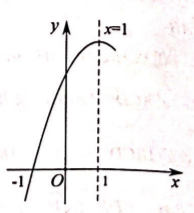①$a b c < 0$;
②$4 a+2 b+c < 0$;
③$8 a+c < 0$;
④若抛物线经过 点 $(-3, n)$, 则关于 $x$ 的一元二次方程 $a x^2+b x+c-n=0(a \neq 0)$ 的两根分别为 $-3,5$.$\text{A.}$ 1个 $\text{B.}$ 2个 $\text{C.}$ 3个 $\text{D.}$ 4个
【答案】 C